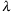The G3GRID Procedure

# GRID Statement

Specifies the three numeric variables for interpolation or for smoothing. Can also specify the number of observations (x and y values), in the output data set; output values for the two horizontal variables x-y; and the interpolation method for the vertical variables.
 Requirements: Exactly one grid request is required.

### Syntax

 GRID grid-request ;
grid-request must be:
 y*x=z(s)

option(s) can be one or more options from any or all of the following categories:

• grid options:

 AXIS1=ascending-value-list AXIS2=ascending-value-list NAXIS1=n NAXIS2=n
• interpolation options:

 JOIN NEAR=n PARTIAL SCALE | NOSCALE SMOOTH=ascending-value-list SPLINE

y*x=z(s)

specifies three or more numeric variables from the input data set:

y

is one of the variables that forms the horizontal (x-y) plane

x

is another of the variables that forms the horizontal (x-y) plane

z(s)

is one or more of the vertical variables for the interpolation

Although the GRID statement can specify only two horizontal variables, it can include multiple vertical variables. Separate vertical variables with blanks:

`grid x*y=z w u v;`

AXIS1=ascending-value-list

specifies a list of numeric values to assign to the first (y) variable in the grid request for the output data set. Numbers that you specify with this option determine the number of values for y, and override a value that you specify with the NAXIS1= option. The ascending-value-list must be arranged in ascending order. The value list can be in any of the following forms:

• n <...n>

• n TO n <BY increment>

• n <...n> TO n <BY increment > <n <...n> >

 Featured in: Using the Default Interpolation Method and Spline Interpolation.
AXIS2=ascending-value-list

specifies a list of numeric values to assign to the second (x) variable in the grid request for the output data set. Numbers that you specify with this option determine the number of values for x and override a value that you specify with the NAXIS2= option. The ascending-value-list must be arranged in ascending order. The value list can be in any of the following forms:

• n <...n>

• n TO n <BY increment>

• n <...n> TO n <BY increment > <n <...n> >

 Featured in: Using the Default Interpolation Method and Spline Interpolation.
JOIN

uses a linear interpolation within a set of triangular regions that are formed from the input data set. This interpolation method creates values in the range of the initial values of the vertical variable, but the resulting interpolated surface might not be smooth.

NAXIS1=n

specifies the number of values for the first (y) variable in the grid request for the output data set. You can determine the actual values used for y by taking the minimum and the maximum values of y and dividing the range into n- one equal sections.

A value specified with NAXIS1= is ignored if values are also specified with AXIS1=.

 Default: 11
NAXIS2=n

specifies the number of values for the second (x) variable in the grid request for the output data set. You can determine the actual values that are used for x by taking the minimum value and the maximum value of x, and dividing the range into n- one equal sections.

A value specified with NAXIS2= is ignored if values are also specified with AXIS2=.

 Default: 11
NEAR=n

specifies the number of the nearest data points to use for computing the estimates of the first derivative, and the second derivative. As NEAR= values become larger, time and computation costs increase significantly. NEAR= is ignored if you specify SPLINE. The value of n must be greater than or equal to 3.

If the number of input data points is insufficient for the number that you specify with NEAR=, a smaller number of data points is used.

 Default: 3 Featured in: Partial Spline Interpolation.
NOSCALE

specifies that the x and y variables not be scaled to the same range before interpolation. By default, the interpolation is performed after both variables are similarly scaled because the interpolation methods assume that the scales of x and y are comparable.

 Default: SCALE
PARTIAL

specifies that a spline be used to estimate the derivatives for the biquintic polynomial interpolation. A bivariate spline is fit to the nearest neighbors, and is used to estimate the needed derivatives. This option produces results that are less smooth than those produced by the SPLINE option and uses fewer computer resources. However, the results produced by PARTIAL are smoother than those that are produced by the default. If you use both the PARTIAL option and the SPLINE option, the PARTIAL option is ignored.

 Featured in: Partial Spline Interpolation.
SCALE

specifies that the x and y variables be scaled to the same range before interpolation. The interpolation is performed after both variables are similarly scaled because the interpolation methods assume that the scales of x and y are comparable.

 Default: SCALE
SMOOTH=ascending-value-list

specifies a list of numbers for smoothing parameters. Use the SMOOTH= option only when you also use the SPLINE option. The ascending-value-list must be arranged in ascending order. The value list can be in any of the following forms:

• n <...n>

• n TO n <BY increment>

• n <...n> TO n <BY increment > <n <...n> >

For each valueof the smoothing parameter, a function u (x, y) is formed that minimizeswhere n is the number of data points, and the pairs (xj, yj ) are the available points, with corresponding function values zj (Wahba 1990).

The higher the value of the smoothing parameter, the smoother the resulting interpolation. The lower the smoothing parameter, the closer the resulting surface is to the original data points. A smoothing parameter of 0 produces the same results as the SPLINE option without the SMOOTH= option.

This procedure repeats for each value of the smoothing parameter. The output data set that you specify in the OUT= option contains:

• the interpolated values

• the values of the grid points

• the values of the smoothing parameter in the variable _SMTH_

• a separate grid for each value of the smoothing parameter

 Featured in: Spline and Smoothing Interpolations.
SPLINE

specifies the use of a bivariate spline (Harder and Desmarais 1972, Meinguet 1979, Green and Silverman 1994) to interpolate, or to form a smoothed estimate, if you also use the SMOOTH= option. The SPLINE option results in the use of an order n3 algorithm, where n is the number of input data points. Consequently, this method can be time-consuming. If you use more than 100 input points, the procedure can use excessive time.

 Featured in: Spline and Smoothing Interpolations and Spline Interpolation.

The G3GRID procedure produces a data set with 121 observations for combinations of eleven values for each of the horizontal variables, x and y. To create a data set with a different number of observations, use the GRID statement's NAXIS1= option, or the NAXIS2= option to specify the number of the values of y or x, respectively. You can use the GRID statement's AXIS1= option or the AXIS2= option to specify the actual values for y or x, respectively.

The following table shows the number of observations that will be in the output data set if you use any of these options.

If you specify multiple smoothing parameters, the number of observations in the output data set will be the number shown in the table, multiplied by the number of smoothing values that you specify in the SMOOTH= option. If you use BY-group processing, multiply the number in the table by the number of BY groups.

Number of Observations Contained in the Output Data Set
Options Specified Number of Observations in Output Data Set
None 121
AXIS1= (number of values for AXIS1=) * 11
AXIS2= (number of values for AXIS2=) * 11
NAXIS1= (value of NAXIS1=) * 11
NAXIS2= (value of NAXIS2=) * 11
AXIS1=, AXIS2= (number of values for AXIS1=) * (number of values for AXIS2=)
AXIS1=, NAXIS1= (number of values for AXIS1=) * 11
AXIS1=, NAXIS2= (number of values for AXIS1=) * (value of NAXIS2=)
AXIS2=, NAXIS1= (number of values for AXIS2=) * (value of NAXIS1=)
AXIS2=, NAXIS2= (number of values for AXIS2=) * 11
NAXIS1=, NAXIS2= (value of NAXIS1=) * (value of NAXIS2=)

Depending on the shape of the original data, and the options that you specify, the output data set can contain values for the vertical (z) values that are outside of the range of the original values in the data set.Previous Page | Next Page | Top of Page